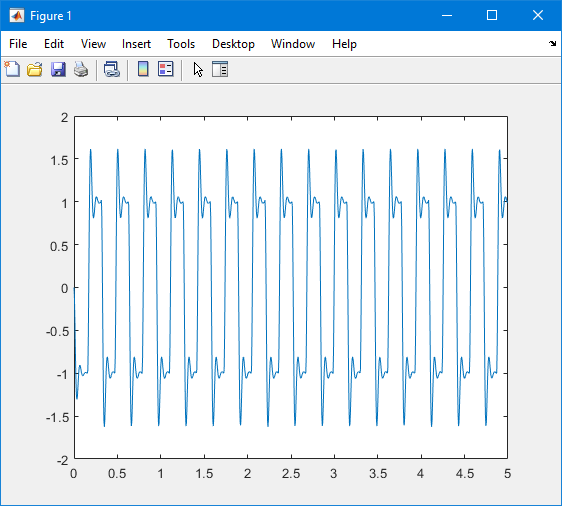## Plot Signal Data Logged to Workspace by Simulink Desktop Real-Time

To visualize non-real-time simulated data or real-time application data, use the MATLAB® plotting functions.

After running your real-time application and logging data to the MATLAB workspace, you can plot the data.

This procedure uses the model `sldrtex_model`. To open this model, in the MATLAB Command Window, type:

```open_system(docpath(fullfile(docroot,'toolbox',... 'sldrt','examples','sldrtex_model')))```

The procedure assumes that you saved your data to the variable `ScopeData`.

The steps in this procedure assume that you have already loaded that model and have completed the steps in Set Scope Parameters for Logging to Workspace and Set Run in Kernel Mode Properties for Logging to Workspace.

1. In the Simulink® Editor, on the Desktop Real-Time tab, click Run in Real Time.

When the real-time application runs, it creates the `ScopeData` variable that you configured in Set Run in Kernel Mode Properties for Logging to Workspace.

2. To show the structure of the variable `ScopeData`, in the MATLAB Command Window, type:

```ScopeData ```
```ScopeData = struct with fields: time: [2001×1 double] signals: [1×1 struct] blockName: 'sldrtex_model/Scope'```

To list the contents of the structure `signals`, type:

```ScopeData.signals ```
```ans = struct with fields: values: [2001×1 double] dimensions: 1 label: '' title: '' plotStyle: 0```
3. To plot the first `1000` points, type:

```plot(ScopeData.time(1:1000),ScopeData.signals.values(1:1000)) ```

The MATLAB environment plots the first `1000` samples over `0.0000–0.9990` seconds.4. The variable `ScopeData` is not automatically saved to your hard disk. To save the variable `ScopeData`, type:

`save ScopeData`

The MATLAB environment saves the scope data to the file `ScopeData.mat`.